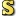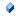﻿NSRect StructureMonobjc Documented Class LibraryNSRect StructureNamespaces ► Monobjc.Foundation ► NSRectC#Visual BasicVisual C++
Represents a rectangle.Declaration Syntax
 C# Visual Basic Visual C++
`public struct NSRect : IEquatable<NSRect>`
```Public Structure NSRect _
Implements IEquatable(Of NSRect)```
`public value class NSRect : IEquatable<NSRect>`Members
 All Members Constructors Methods Properties Fields PublicProtected InstanceStatic DeclaredInherited
IconMemberDescriptionNSRect(Single, Single, Single, Single)
Initializes a new instance of the NSRect class.NSRect(NSPoint, NSSize)
Initializes a new instance of the NSRect class.CGRect
Converts this instance to a CGRectContainsRect(NSRect)
Returns a Boolean value that indicates whether this rectangle completely encloses another.DivideRect(NSRect%, NSRect%, Single, NSRectEdge)
Divides this rectangle into two new rectangles.Equality(NSRect, NSRect)
Implements the operator ==.Equals(NSRect)
Equalses the specified ns rect.Equals(Object)
Indicates whether this instance and a specified object are equal.
(Overrides ValueType.Equals(Object).)Finalize()()()()
Allows an Object to attempt to free resources and perform other cleanup operations before the Object is reclaimed by garbage collection.
(Inherited from Object.)GetHashCode()()()()
Returns the hash code for this instance.
(Overrides ValueType.GetHashCode()()()().)GetType()()()()
Gets the Type of the current instance.
(Inherited from Object.)Height
Returns the height of this rectangle.Inequality(NSRect, NSRect)
Implements the operator !=.InsetRect(Single, Single)
Creates a rectangle that insets this rectangle by a specified amount.IntegralRect()()()()
Creates a rectangle with the sides of this rectangle to integer values.IntersectionRect(NSRect)
Calculates the intersection of this rectangle with the specified rectangle.IntersectsRect(NSRect)
Returns a Boolean value that indicates whether this rectangle and the specified rectangle intersect.IsEmptyRect
Returns a Boolean value that indicates whether this rectangle is empty.MaxX
Returns the largest x coordinate of the rectangle.MaxY
Returns the largest y coordinate of the rectangle.MemberwiseClone()()()()
Creates a shallow copy of the current Object.
(Inherited from Object.)MidX
Returns the x coordinate of the rectangle�s midpoint.MidY
Returns the y coordinate of the rectangle�s midpoint.MinX
Returns the smallest x coordinate of the rectangle.MinY
Returns the smallest y coordinate of the rectangle.MouseInRect(NSPoint, Boolean)
Returns a Boolean value that indicates whether the point is in the rectangle.NSContainsRect(NSRect, NSRect)
Returns a Boolean value that indicates whether one rectangle completely encloses another.NSDivideRect(NSRect, NSRect%, NSRect%, Single, NSRectEdge)
Divides a rectangle into two new rectangles.NSEqualRects(NSRect, NSRect)
Returns a Boolean value that indicates whether the two rectangles are equal.NSHeight(NSRect)
Returns the height of a given rectangle.NSInsetRect(NSRect, Single, Single)
Insets a rectangle by a specified amount.NSIntegralRect(NSRect)
Adjusts the sides of a rectangle to integer values.NSIntersectionRect(NSRect, NSRect)
Calculates the intersection of two rectangles.NSIntersectsRect(NSRect, NSRect)
Returns a Boolean value that indicates whether two rectangles intersect.NSIsEmptyRect(NSRect)
Returns a Boolean value that indicates whether a given rectangle is empty.NSMakeRect(Single, Single, Single, Single)
Creates a new NSRect from the specified values.NSMaxX(NSRect)
Returns the largest x coordinate of a given rectangle.NSMaxY(NSRect)
Returns the largest y coordinate of a given rectangle.NSMidX(NSRect)
Returns the x coordinate of a given rectangle�s midpoint.NSMidY(NSRect)
Returns the y coordinate of a given rectangle�s midpoint.NSMinX(NSRect)
Returns the smallest x coordinate of a given rectangle.NSMinY(NSRect)
Returns the smallest y coordinate of a given rectangle.NSMouseInRect(NSPoint, NSRect, Boolean)
Returns a Boolean value that indicates whether the point is in the specified rectangle.NSOffsetRect(NSRect, Single, Single)
Offsets the rectangle by the specified amount.NSPointInRect(NSPoint, NSRect)
Returns a Boolean value that indicates whether a given point is in a given rectangle.NSRectFromCGRect(CGRect)
Converts a CGRect instance to a NSRectNSRectFromString(NSString)
Returns a rectangle from a text-based representation.NSRectToCGRect(NSRect)
Converts a NSRect instance to a CGRectNSStringFromRect(NSRect)
Returns a string representation of a rectangle.NSUnionRect(NSRect, NSRect)
Calculates the union of two rectangles.NSWidth(NSRect)
Returns the width of the specified rectangle.NSZeroRect
A NSRect with its origin and size set to zero.OffsetRect(Single, Single)
Creates a rectangle that offsets this rectangle by the specified amount.origin
The origin of the rectangle (its starting x coordinate and y coordinate).PointInRect(NSPoint)
Returns a Boolean value that indicates whether a given point is in a specified rectangle.size
The width and height of the rectangle, as measured from the origin.ToString()()()()
Returns the a string representation of this instance.
(Overrides ValueType.ToString()()()().)UnionRect(NSRect)
Creates a rectangle as the union of this rectangle and the specified rectangle.Width
Returns the width of the rectangle.Version Information
• Available in Monobjc Bridge: 10.6 (For Mac OS X 10.6 and later), 10.5 (For Mac OS X 10.5 and later)

Assembly: Monobjc.Foundation (Module: Monobjc.Foundation)